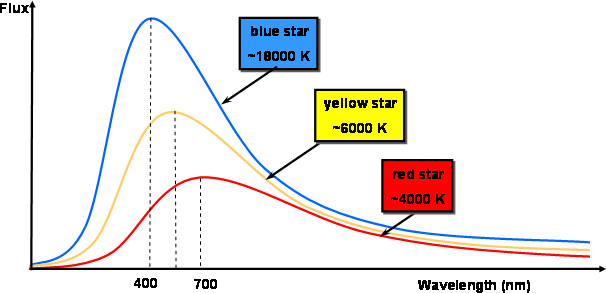# Solar System Energy

Updated 2019-09-18

Planetary energy balance:

## Blackbody Emission

There are two conditions required for blackbody radiation:

1. The body must be in thermal equilibrium - has a well-defined temperature T

2. The body is dense/opaque such that photos can’t simply pass through it but instead bounce around many times on the way to exiting.

The total flux of blackbody radiation is given by: $$F=\sigma T^4\quad[\text{Js}^{-1}\text{m}^{-2}]$$ Where $\sigma$ is the Stefan-Boltzmann constant which is 5.56×10-8 Js-1m-2K-4.

For humans, our body is at 310 K, objects radiate about 500Wm-2 where 1W is 1Js-1. If the average human surface area is 2m2 then we radiate about 1000W.

The surface of the Sun is around 5780K. Which radiates about 6.3×106 Wm-2.

Now we looked at the total flux, it’s actually an integral of the intensity for all wavelengths: $$F=\sigma T^4 = \pi\int_0^\infty I_\lambda \mathrm d\lambda$$ Where $I_\lambda$ is the intensity per unit wavelength.Temperature is the only parameter needed to describe the blackbody radiation.